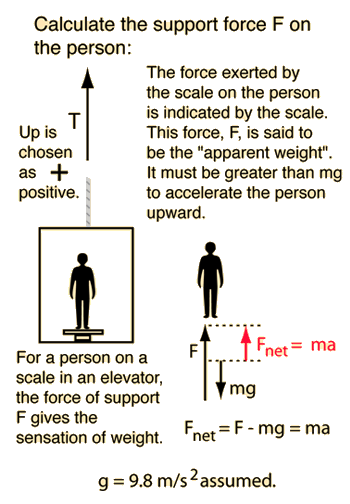# Elevator Problem

This is an application of Newton's second law to the forces felt in an elevator. If you are accelerating upward you feel heavier, and if you are accelerating downward you feel lighter. If the elevator cable broke, you would feel weightless since both you and the elevator would be accelerating downward at the same rate.

### support force F = mass x acceleration + weightFor a mass m= kg, the elevator must support its weight = mg =Newtons to hold it up at rest. If the acceleration is a=m/s² then a net force=Newtons is required to accelerate the mass. This requires a support force of F=Newtons. Note that the support force is equal to the weight only if the acceleration is zero, and that if the acceleration is negative (downward), the support force is less than the weight. If you enter a downward acceleration greater than 9.8 m/s² you will get a negative support force, showing that you must force it downward to get an acceleration greater than that of free fall.

### See lifting mass problem

 "Weightless" condition if the cable breaks.
Index

Newton's laws

Standard mechanics problems

 HyperPhysics***** Mechanics R Nave
Go Back

# You Feel "Weightless" If the Elevator Cable BreaksThe phenomenon of "weightlessness" occurs when there is no force of support on your body. When your body is effectively in "free fall", accelerating downward at the acceleration of gravity, then you are not being supported. The sensation of apparent weight comes from the support that you feel from the floor, from a chair, etc. Different sensations of apparent weight can occur on an elevator since it is capable of zero or constant speed (zero acceleration) and can accelerate either upward or downward. If the elevator cable breaks then both you and the elevator are in free fall. The resultant experience of weightlessness might be exhilirating if it weren't for the anticipation of the quick stop at the bottom.

 Elevator calculation
Index

Newton's laws

Standard mechanics problems

 HyperPhysics***** Mechanics R Nave
Go Back